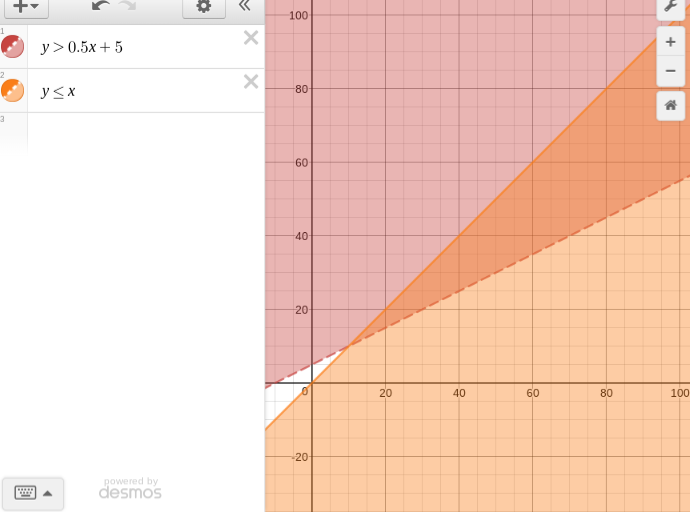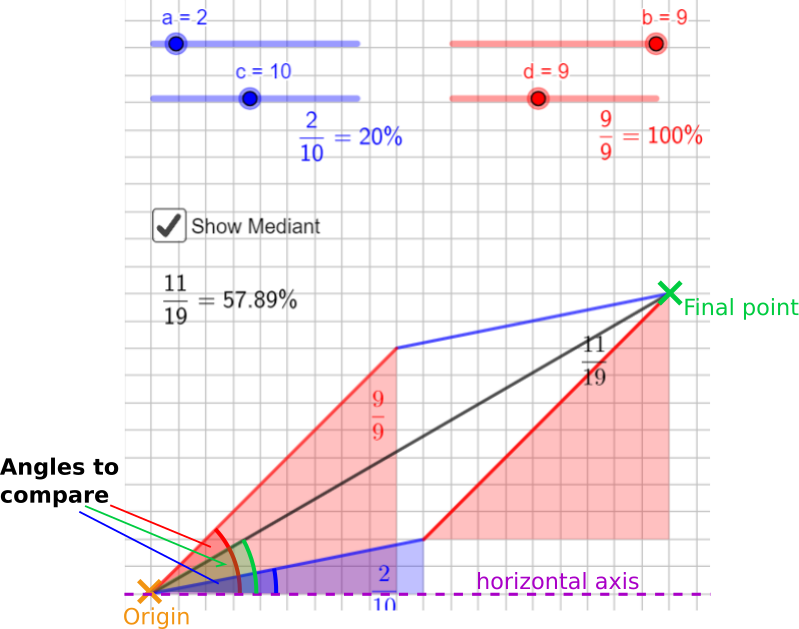#### You may also likeA 1 metre cube has one face on the ground and one face against a wall. A 4 metre ladder leans against the wall and just touches the cube. How high is the top of the ladder above the ground?In this problem we are faced with an apparently easy area problem, but it has gone horribly wrong! What happened?### From All Corners

Straight lines are drawn from each corner of a square to the mid points of the opposite sides. Express the area of the octagon that is formed at the centre as a fraction of the area of the square.

##### Age 14 to 16Challenge Level

Pradeep from Peak School in Hong Kong and Vicente José from Kings College Alicante in Spain gave their opinions about the dispute. Pradeep said:
75% on Section 1
35% on Section 2
The mean of these two gives 55% so technically Kyle is right but if you take the overall score of both exams you get 50% so the teacher is right too.

This problems answer depends on how you choose to grade either you take the mean of the scores or take the overall scores.

Vicente José said:
The first question asked in the problem is if we agree with that Kyle only deserves a 50% in her exam. I do agree she only deserves a 50% in her exam as she must have the combined mean, mediant, of her two exams depending on the number of marks awarded on each in order to give more weighting to the one with more marks.

Oliver from The Priory Academy LSST in England and Clara from Bangkok Patana School in Thailand found some examples of when Kyle and the teacher would agree about the overall score. This is Clara's work:
The average and the median marks are the same when the both fractions / percentages are equal. The tests scores where both Kyle and his teacher would agree on his test score would be:
$\frac0{12}$ and $\frac0{20} =0\%$
$\frac3{12}$ and $\frac5{20} =25\%$
$\frac6{12}$ and $\frac{10}{20} =50\%$
$\frac9{12}$ and $\frac{15}{20} = 75\%$
$\frac{12}{12}$ and $\frac{20}{20} = 100\%$

Siddhant from Indus International School of Bangalore in India found some examples of pairs of fractions ($\frac ac$ and $\frac bd$) for which the mean is equal to the mediant.
When I started solving this problem, I realized that there were several, unique solutions to the first part of the problem. For example, one solution I got was:
a = 2
b = 1
c = 8
d = 4
In this case, the averages and the mediant are both 25%.
Another example could be:
a = 4
b = 2
c = 8
d = 4
In this case, the averages and the mediant are both 50%.
What do Siddhant's fractions have in common with what Clara and Oliver noticed?

Vicente José found another way to generate a pair of fractions which have the mediant equal to the mean:
For the mean, $\dfrac{\text{grade A + grade B}}2$, to be the same as the mediant, there are two combinations possible.
1)     $c=d$ and so there are the same number of marks in each test and therefore the same weighting is taken on each and so the mean = mediant
Eg. $\frac{25}{50}$ and $\frac{40}{50}$
Mediant: $\frac{25+40}{50+50} =\frac{65}{100} = 65\%$
Mean: $( \frac{25}{50} + \frac{40}{50} ) \div2 = 1.3\div2 = 65\%$
2)     $\frac ab=\frac cd$ if both fractions are equal the mediant and mean will be the same as the mean and mediant will both be $\frac ab$ which is also $\frac cd$ (as we had already seen).

The pass mark for an exam is 50%. If I scored 25% on the first $n$ questions, under what circumstances can I still pass the exam?
Clara started by looking at different ways to get 25%:

The only results you can get to get $25\%$ are:
$\frac14, \frac28, \frac3{12}, \frac4{16}, \frac5{20},$ and so on.

This is a pattern where the numerator increases by $1$ and the denominator increases by $4$ each time. All of the fractions can be simplified to $\frac14.$

Siddhant began using algebra, but was actually using the sum, not the mediant:
Pass Mark $= 50\%$
$25\%$ of $n$ questions $= 0.25n$
\begin{align}&\tfrac{0.25n}n +\tfrac bd \ge 0.5\\ \Rightarrow & \tfrac{0.25n}n = 0.5 - \tfrac bd\\ \Rightarrow &0.25 = 0.5 = -\tfrac bd\\ \Rightarrow &-0.25 = -\tfrac bd\\ \Rightarrow&\tfrac bd = 0.25\end{align}

The only way that he will pass is if he scores greater than or equal to $25\%$ in his remaining questions.

Vicente José also used algebra:
If $\frac ab = 0.25$ and $b=n$ then $\frac ab = \frac{0.25n}{n}$

If mediant is $\frac{a+b}{c+d}$ and $\frac ab = \frac{0.25n}{n}, \frac cd = \frac yx$ then $\frac{0.25n+y}{n+x}$

If the pass grade is $50\%$ (or $0.5$) then $$\frac{0.25n+y}{n+x}=0.5$$
Multiply by $n+x$: $$0.25n + y = 0.5(n+x)$$ Multiply by $2$: $$0.5n + 2y = n+x$$ Subtract $0.5n$: $$2y = x + 0.5n$$ Divide by two: $$y=0.5x+0.25n$$
The final equation is a linear equation with three unknowns $y, x$ and $n.$ $y$ and $x$ are representative of the next grade obtained, $\frac yx$; and $n$ the number of marks in the first grade.

Vicente José used this to create a graph:
We will then make this an inequality of $y>0.5x+0.25n$, to get all possible answers to make her average score $50\%,$ and we will do another inequality, $y\le x,$ in order to get the real answers as it is impossible to achieve more marks than can be awarded. The area made by the intersection of these will be all possible answers of $\frac cd$ or $\frac yx$ to get a $50\%$ or above.

In the image of the graph you can see the possible answers when the first test was out of $20$ marks.Is it true that the mediant always lies between the two fractions? How do you know?
This is Vicente José's explanation, with a diagram added, which is taken from the vector graph in the question:

This answer is going to be answered in relation to the vector graph given. We know this as when this is put in vector quantities as shown in the question and we add these up, the angle made by the final point to the origin of the vectors and a horizontal imaginary axis will always lie between the first and second vector/grade.